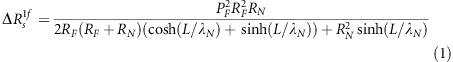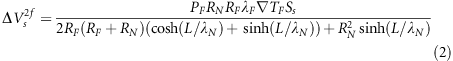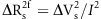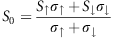## Introduction

Spin current is central to the operation of spin-based nanoelectronic devices.1, 2, 3, 4, 5 The efficient manipulation of spin current is essential for developing functional and energy-efficient nanospintronic devices. Recently, heat has been used as a new approach to control the spin in ferromagnetic/nonmagnetic hybrid nanostructures.6, 7, 8, 9, 10, 11, 12, 13, 14 A representative and fascinating phenomenon is thermal spin injection, in which excess heat can be used to produce spin current because of the spin-dependent Seebeck coefficient.7, 8, 9 Until now, thermally driven spin injection has only been demonstrated using conventional ferromagnetic metals such as permalloy (Py)7 and cobalt.13 However, thermally excited spin current can only generate a few tens of nV which is low, because of the low spin-dependent Seebeck coefficient of conventional ferromagnetic metals.

In electrical spin injection, the difference in the electrical conductivity between the up and down spins produces spin current.4, 5 However, because the direction of movement of the electrons is determined by the electric field, the electrons with up spins move in the same direction as those with down spins. Therefore, the generation efficiency of the spin current cannot exceed 100% even when a fully spin-polarized material, that is, a half-metal, is used. Furthermore, the spin current induced by thermal spin injection is determined by the difference in the Seebeck coefficient between the up and down spins,7, 15 which is known as the spin-dependent Seebeck effect. This effect is different from the spin Seebeck effect,6, 14 although both effects produce spin current and spin accumulation from the temperature gradient. The Seebeck coefficient is strongly correlated to the band structure around the Fermi level, and under simple approximation in metals, the coefficient is proportional to the energy derivative of the logarithmic density of state (DOS) at the Fermi level.16, 17, 18 In a ferromagnetic material, because the DOS has different features for up and down spins, one must separately consider the movement directions of the up-spin electrons and the down-spin electrons.

For conventional ferromagnets, the difference in the band structure between the up and down spins can be approximated as a small shift in the DOS. In this case, although the up-spin and down-spin electrons produce a Seebeck effect of a different magnitude, the difference is small. Moreover, electrons of either spin diffuse in the same direction, as shown in Figures 1a and b. Therefore, the magnitude of the spin current diminishes. If a ferromagnetic material has a large difference in the DOS between up and down spins, a sign reversal of the Seebeck coefficient between the up and down spins may be produced. In this situation, the up-spin and down-spin electrons flow in opposite directions, as shown in Figures 1c and d. Therefore, the generation efficiency of the spin current that occurs because of thermal spin injection is significantly enhanced by the large spin-dependent Seebeck coefficient.

To realize this favorable situation for efficient thermal spin injection, we focused on a ferromagnetic CoFeAl (CFA) alloy, which is expected to have high spin polarization even in a simply evaporated polycrystalline film because of the local formation of a highly spin-polarized Heusler compound.19, 20 This characteristic is an important advantage for preparing multiterminal functional lateral spin valve (LSV) structures because no specific substrate or thermal treatment is required during the sample fabrication process.

## Experimental procedure

Our CFA film was deposited using electron-beam evaporation from a CFA melting source, whose composition was 48 wt% Co–48 wt% Fe–4 wt% Al. The composition of the evaporated film and its uniformity were confirmed using an energy dispersive X-ray detector in a scanning electron microscope system. The obtained composition of the evaporated film was 43 wt% Co–54 wt% Fe–3 wt% Al from the energy dispersive X-ray analysis. It should be noted that the Al content was considerably different from the previously reported values.19, 20

A batch of conventional LSV structures with two CFA wires bridged by a nonmagnetic Cu wire was fabricated on a SiO2/Si substrate using electron beam lithography and the lift-off technique. Figure 2a shows a scanning electron microscope image of a representative device in our study. First, two CoFeAl wires, each of which was 30 nm thick and 120 nm wide, were prepared using an electron beam evaporator with a base pressure of approximately 10−9 torr. We introduced a difference in the edge shapes between the two wires to easily control the magnetization configuration using an external magnetic field. The interval distances (L), which correspond to the center–center distance between the two wires, were varied from 200 to 1500 nm on the same chip. Then, the Cu channel wires, each of which was 160 nm thick and 120 nm wide, were deposited using Joule heating evaporation. The surfaces of the CoFeAl wires were carefully cleaned using low-voltage Ar ion milling before depositing Cu to obtain a highly transparent interface (see Supplementary Information). The resistivity of the CFA wires was evaluated from the four terminal resistance of a well-defined wire in the same batch and estimated to be 4.5±0.2 × 10−7 Ωm at room temperature, which is one order of magnitude smaller than the previously reported value. Because the present value is close to the value for CoFe alloy,20 the difference in resistivity is most likely because of the difference in Al content. The electrically and thermally driven pure spin currents were detected using a low-frequency lock-in technique with the first- and second-harmonic voltage responses, respectively.

## Results and discussion

First, we evaluated the electrical spin polarization of the CFA wires by measuring the nonlocal spin signal under the electrical spin injection, as shown in Figure 2a. The electric current across the ferromagnetic/nonmagnetic interface creates spin accumulation in the nonmagnetic Cu channel. This nonequilibrium spin accumulation produces a spin current without an accompanying charge current, that is, a pure spin current, on the upside of the nonmagnetic channel.5, 21, 22 The pure spin current can be detected using another ferromagnetic electrode after it propagates in the nonmagnetic channel. As shown in Figure 2b, the nonlocal electrical voltage shows a clear spin valve signature with an overall resistance change of more than 10 mΩ at room temperature. For comparison, the spin signal for a similarly fabricated Py/Cu LSV with the same device dimensions is shown in Figure 2b. We confirmed that the spin signal for the CFA/Cu LSV was 10 times larger than that for the Py/Cu LSV, which indicates the superior properties of our developed CFA injector.

To elucidate the main mechanism of the significant enhancement of spin signal in the CFA/Cu LSV, we measured the interval dependence of the spin signals for both CFA/Cu and Py/Cu LSVs. As shown in Figure 2c, the dependences of the spin signal on the interval L for both devices were well fitted using the one-dimensional spin diffusion equation with transparent interfaces, which is given by23, 24, 25where PF is the spin polarization of the ferromagnet, λN is the spin diffusion length of the nonmagnet and RF and RN are the spin resistances of the ferromagnet and nonmagnet, respectively. The spin resistance Rs is defined as 2ρλ/(A(1−P2)), where P, ρ and λ are the spin polarization, electrical resistivity and spin diffusion length, respectively. For a nonmagnet, P should be zero. A is the effective cross-section of the spin current. For the ferromagnet, because the spin diffusion length is short, the effective cross-section is given by the area of the ferromagnetic/nonmagnetic junction.

It should be noted that the estimated spin diffusion length of Cu from the fittings for both CFA/Cu and Py/Cu LSVs was 450 nm. If we assume that CFA and Py have identical spin diffusion lengths of 2 nm,25 the spin polarizations of CFA and Py can be estimated as 0.62 and 0.36, respectively. Thus, the strong enhancement of the spin signal by a factor of 10 is attributed to the efficient generation and detection of the spin current because CFA has a large spin polarization and the spin current backflow is significantly reduced.26 The obtained spin polarization of the present CFA is close to the previously reported values, although the Al content and electrical resistivity are notably different,19, 20 which suggests that our CFA has a different crystal structure from those in the previous reports.

Then, we performed thermal spin injection by changing the probe configuration in the same device. To create a temperature gradient across the ferromagnetic/nonmagnetic interface, which is the driving force of thermal spin injection, a large AC charge current flowed in the ferromagnetic wire, as shown in Figure 3a. From a numerical simulation based on COMSOL, a large temperature gradient around the interface was effectively produced by the large current flow in the ferromagnetic injector, as shown in Figure 3b.7, 27 Because of the low resistivity of Cu, a partial current flowing in the ferromagnetic injector was injected into the Cu around the junction, which might contribute to the spin accumulation using electrical spin injection. However, such contributions were excluded using the second-harmonic lock-in technique. In addition, the contribution of the electrical spin injection was confirmed to be negligible by the first-harmonic measurement (see Supplementary Information).

We measured the second-harmonic voltage under the large AC current flow as a function of the magnetic field in the CFA/Cu LSV. As seen in Figure 3c, the voltage clearly reflected the relative angle between the spin injector and the detector, which was similar to the spin signal under the electrical spin injection. We performed similar measurements using the Py/Cu LSV with identical device dimensions. The signal was dominated by the anomalous Nernst–Ettinghausen effect in the Py detector, although a tiny spin signal with a magnitude of 7 nV was observed.28

We also confirmed that the voltage difference between the parallel and antiparallel states parabolically increased with the increase in AC bias current, as shown in Figure 3d. In addition, the interval dependence of the voltage difference is well explained using the following equation, which was obtained from the one-dimensional spin diffusion model (see Supplementary Information):where TF is the temperature gradient in the ferromagnet around the interface and Ss is the spin-dependent Seebeck coefficient of the ferromagnetic injector. The parameters obtained in the electrical spin injection experiments are consistent with the thermal spin injection experimental results. These characteristics are clear evidence of thermal spin injection. As shown in Figure 3e, by fitting the diffusion function to the interval dependence of the thermal spin signal, which is defined as, with the COMSOL simulation results (TF=64 K μm−1, I=0.78 mA), we obtained −72.1 μV K−1 as the spin-dependent Seebeck coefficient for the CFA (see Supplementary Information). This value is 20 times larger than the previously reported value in Slachter et al.,7 which implies that our CFA is a highly efficient thermal spin generator.

We can also estimate the effective Seebeck coefficient S0 from the second-harmonic voltage background.29 The background voltage parabolically increased with increasing bias AC current. From the fitting with the assumption, we obtained a conventional Seebeck coefficient of −22 μV K−1. Notably, the spin-dependent Seebeck coefficient was larger than the conventional Seebeck coefficient. From the relationships ofand Ss=SS, the calculated Seebeck coefficients for up-spin (S) and down-spin (S) electrons were −35.7 and 36.4 μV K−1, respectively. Thus, the spin-dependent Seebeck coefficient was significantly enhanced because of the sign reversal of the Seebeck coefficient. We note that some of the band-structure calculations show that the energy derivatives for the DOSs around the Fermi level has opposite signs for up and down spins.30, 31 Understanding the correlation between the electrical spin polarization and the spin-dependent Seebeck coefficient may be important for further development of thermal spin injection and may provide a relationship between the spin-dependent Seebeck effect and the spin Seebeck effect.32 Systematic experiments for various ferromagnetic alloys with further development of the band-structure calculations will be indispensable to find more excellent materials for the thermal spin injector.

## Conclusion

The temperature gradient that is the driving force of thermal spin injection can be produced using direct physical contact with a hot object, laser irradiation or microwave absorption. Efficient spin current generation using thermal spin injection provides unique architectures for wireless spintronic devices, which greatly simplifies device integration.33 In addition, from the energy-harvesting viewpoint, heat usage is one of the most promising approaches for clean-energy technology. The discovery of excellent materials for thermal spin injection opens a new path for spintronic-device application.